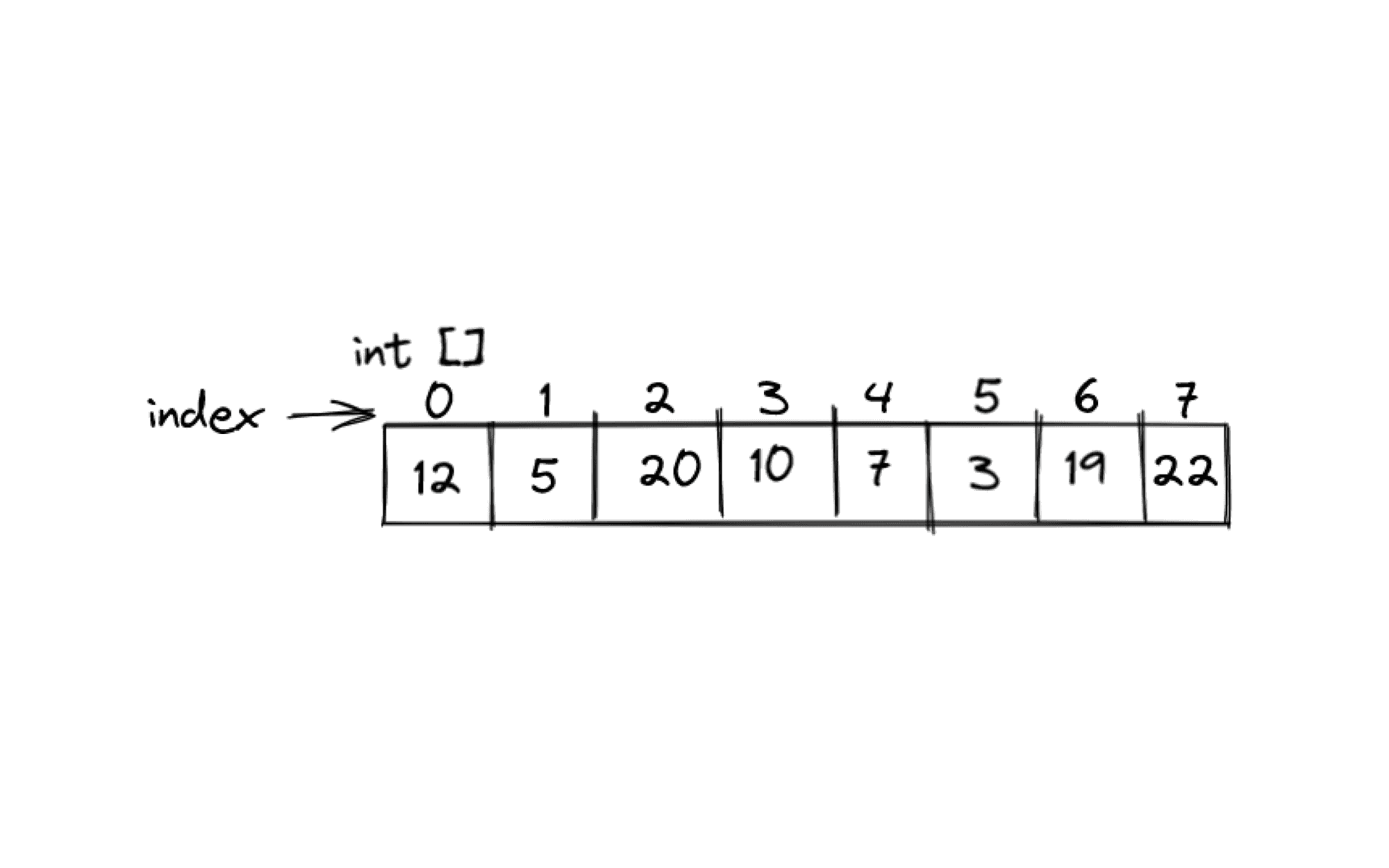We launched our first course, Introduction to Data Structures in C++! Learn more 🎉

Data Structures

# Introduction to Arrays in C++

July 6, 2022Arrays are one of the most commonly used Data Structures while programming. I’m sure you must have seen it and used it before in one form or the other without even knowing it is called an array.

A formal understanding is necessary to use it properly though. Let’s learn about what an array is and how to implement it in C++ 🌟

### Why do we need arrays?

Before we try to understand what an array is, let’s understand why do we even need an array.

Say you are given the job to store roll numbers of all the students in your classroom. If there’s only one student, you would store it as:

int roll_1 = 23;

But what if there are 500 students in your classroom? Would you keep writing int roll_n = number 500 times? That’s too much effort right?

To solve this issue, arrays are used. Now let’s learn about what arrays are.### What is an array?

An array is basically a collection of items that belong to the same data type. Formally, it is a linear data structure which stores data items of the same type.

These data items are stored linearly or in consecutive memory locations in the computer.

Every data item in an array starts storing data items from index ‘0’ up to index ‘N-1’, where ‘N’ is the size of the array. For example in the image below, the size of the array ‘arr’ is 5. Here ‘N’ is the size of array which is 5. Therefore the indices of the array go from 0 to 4 where:

arr = 4;

arr = 6;

arr = 2;

arr = 5;

and arr = 9;### Declaration and Initialisation of an array

Before we get into how to declare and initialize an array, let us understand the difference between the two.

Declaration is like adding a new row in your classroom, while initialisation is like assigning students to the existing seats in a row.

Since we know what the difference is now, let’s understand how to actually declare and initialise an array in C++.

The syntax for declaring an array in C++ is:

data_type name_of_array[size];

where:

data_type is the type of data that will be stored in the array (int, char, etc.);

name_of_array is the name that you give to the array;

and size is the size of the array that has to be defined beforehand;

For an example, if I have to declare an array with name “rows”, data type as “int”, and size as 5, it would be done like this:

int rows;

There’s not just one way to declare an array though. Based on the situation, there are multiple ways in which you can do it.

Situation 1: Declaring an array when you know the size

In this situation, you declare the array as mentioned above.

Example:

int arr1;

This means that we have declared an array of size 10. It can store at most 10 elements of type int.

Situation 2: Declaring an array when you don’t know the size

In this situation, you need to take the size of the array as an input from the user. This is done using the cin operator.

Example:

int n;

cin>>n;

int arr2[n];

C++ allows us to declare an array of user-specified size on run-time where we can use any int variable declared by user as the size of the array.

Once you have declared an array, initialising it is easy. You can initialise an array like this 👇

int arr={1,2,3};

In this example, the elements stored in the array will be 1, 2, and 3 in their respective indices.

### Accessing elements in an array

Accessing elements of an array is one of the most basic tasks but could be a tricky one, so let us understand how we can do it:

As mentioned before, elements are numbered from left to right, starting with 0. The position is referred to as the “index” of the element and we can access any element if we know its index.

int arr={12, 5, 20, 10, 7, 3, 19, 22};If I want to know the number at the 4th position, I can access it as arr. Note that since indexing starts from 0, the index of 1st element is 0, 2nd element is 1, and so on.

arr will give me 10. I can use it as I use any other variable in a program. I can use it in arithmetic operations, print it, or even take input. For example:

int a = arr*arr; //a will have the value 100

cout<<arr<<endl; // this will print 10

cin>>arr; //this takes input from the user and will assign the input to arr

If I want to update the number at the 4th position to 40 we can do it by,

arr=40 // this will place 40 at index 3

This is all about the basics of arrays in C++ 🎉 Once you have learnt the basics, you can learn about the operations that can be performed on arrays and different types of arrays.

Our first course, Introduction to Data Structures in C++ worth ₹499/month is available at a price of your choice for the first month access.

Offer available for a limited time

Subscribe

301/302, 3rd Floor, Saket Callipolis, Sarjapur Main Rd, Doddakannelli, Bengaluru, Karnataka 560035

For any queries, reach out to us at hello@byteavenue.org

For any queries, reach out to us at hello@byteavenue.org

301/302, 3rd Floor, Saket Callipolis, Sarjapur Main Rd, Doddakannelli, Bengaluru, Karnataka 560035